FULL BREAKDOWN OF TIER 4 RIFTS

So I decided to make a guide on all of the probabilities of getting certain rewards. This took me a long time so I hope you find it useful. Enjoy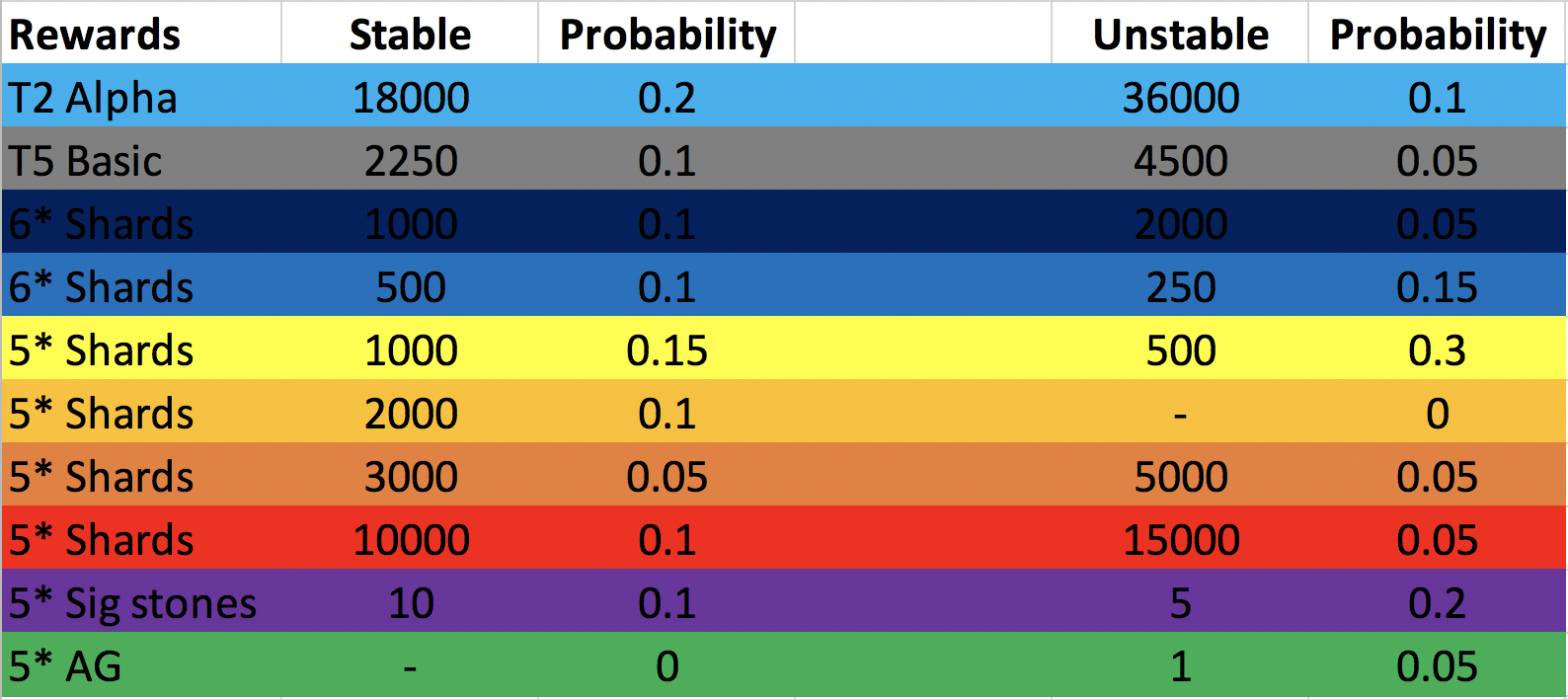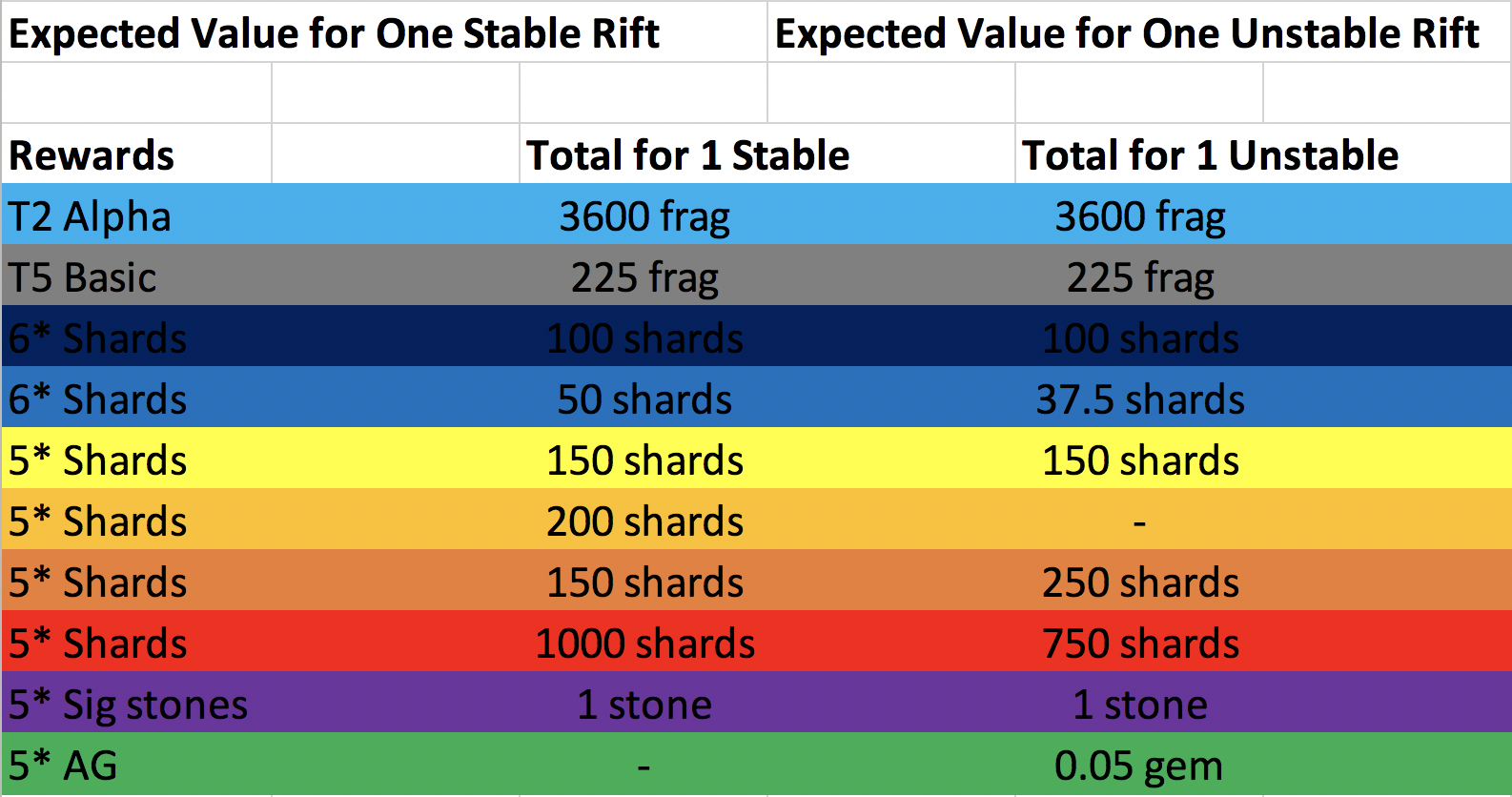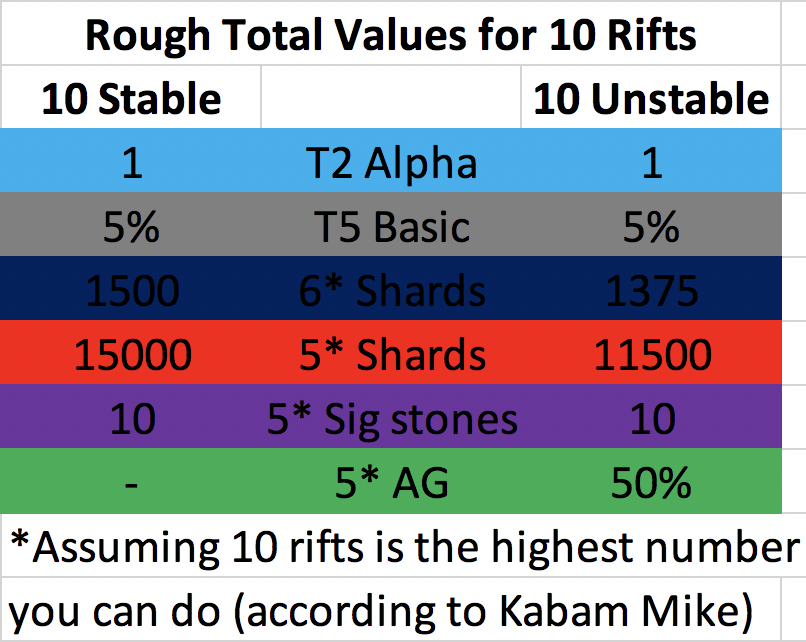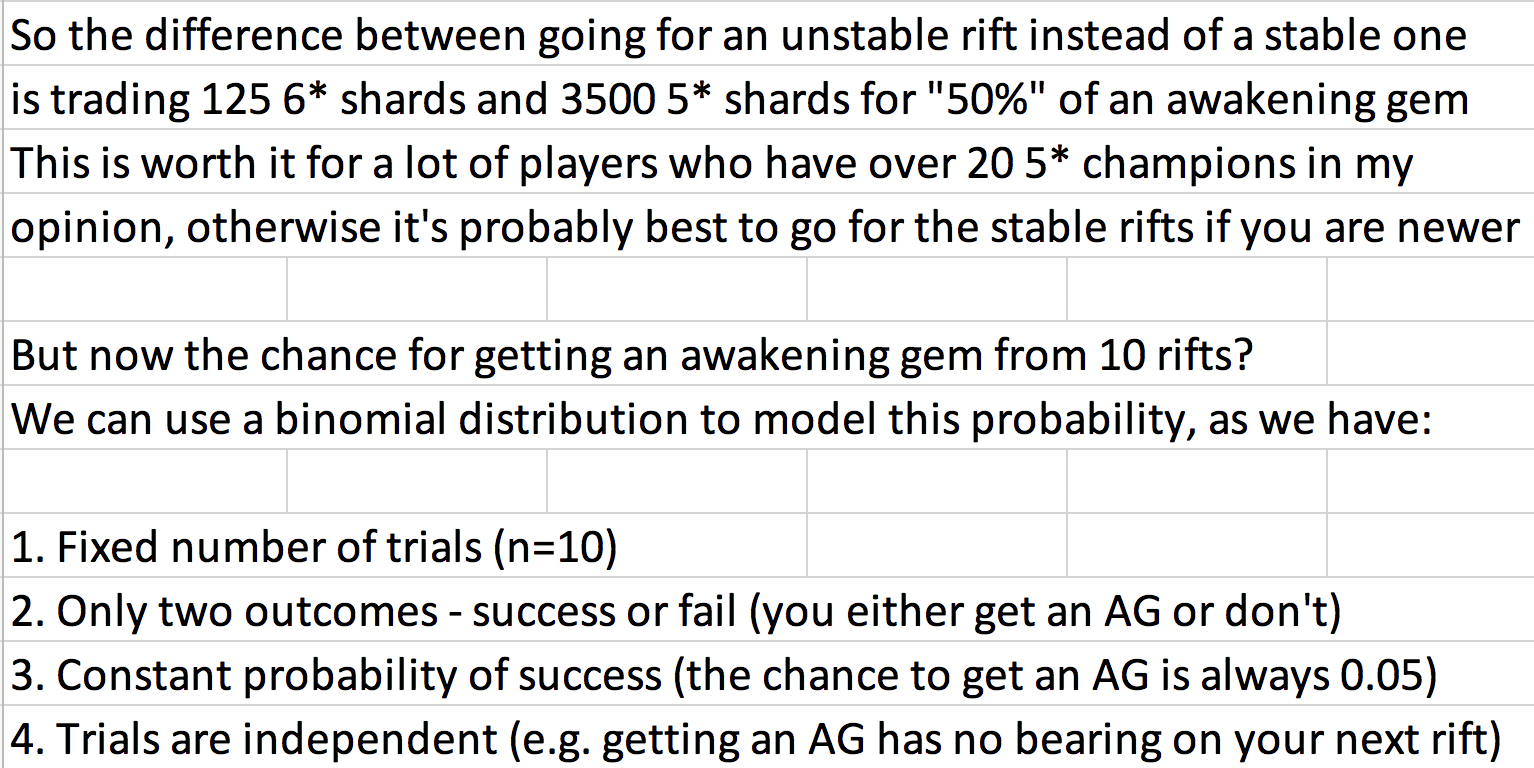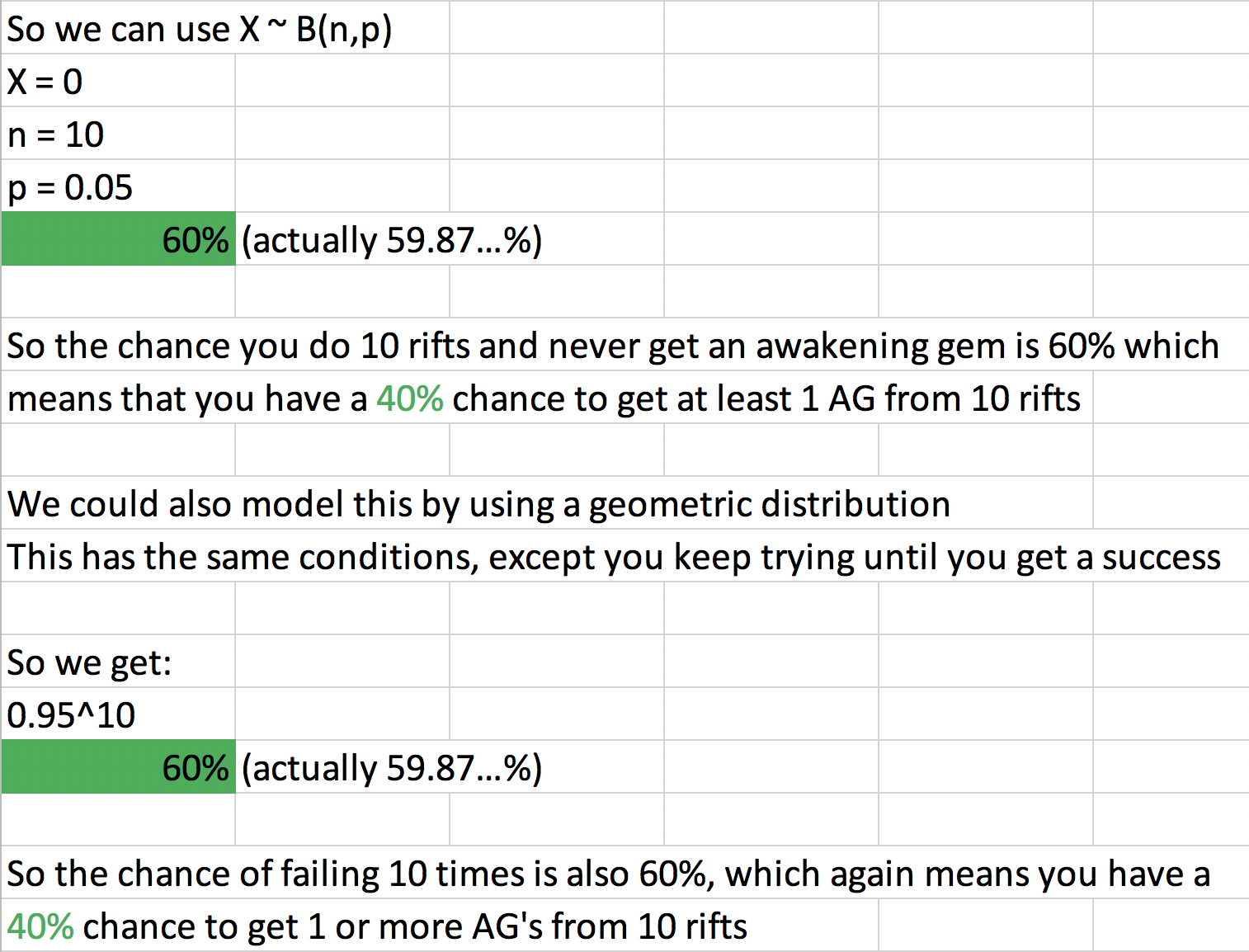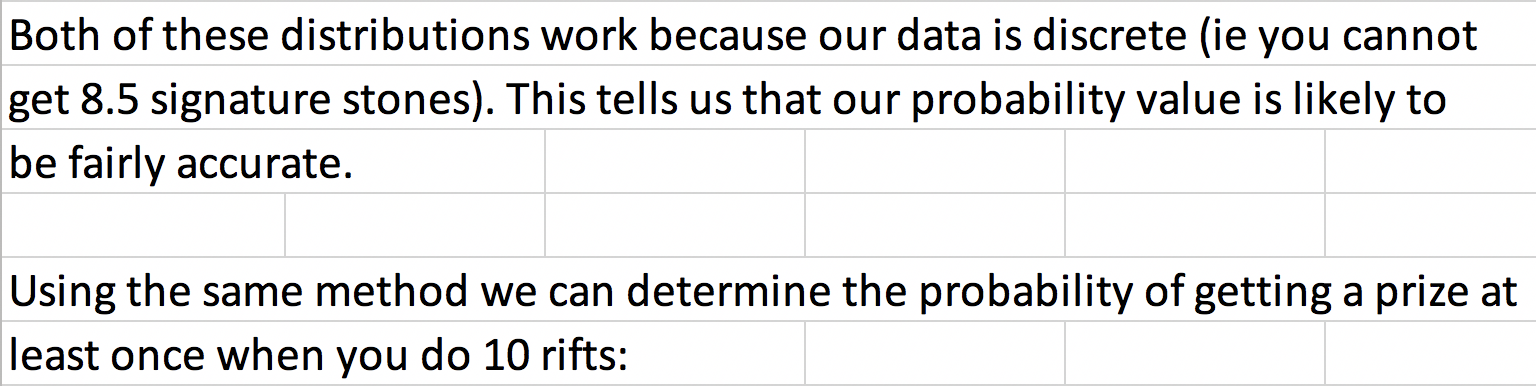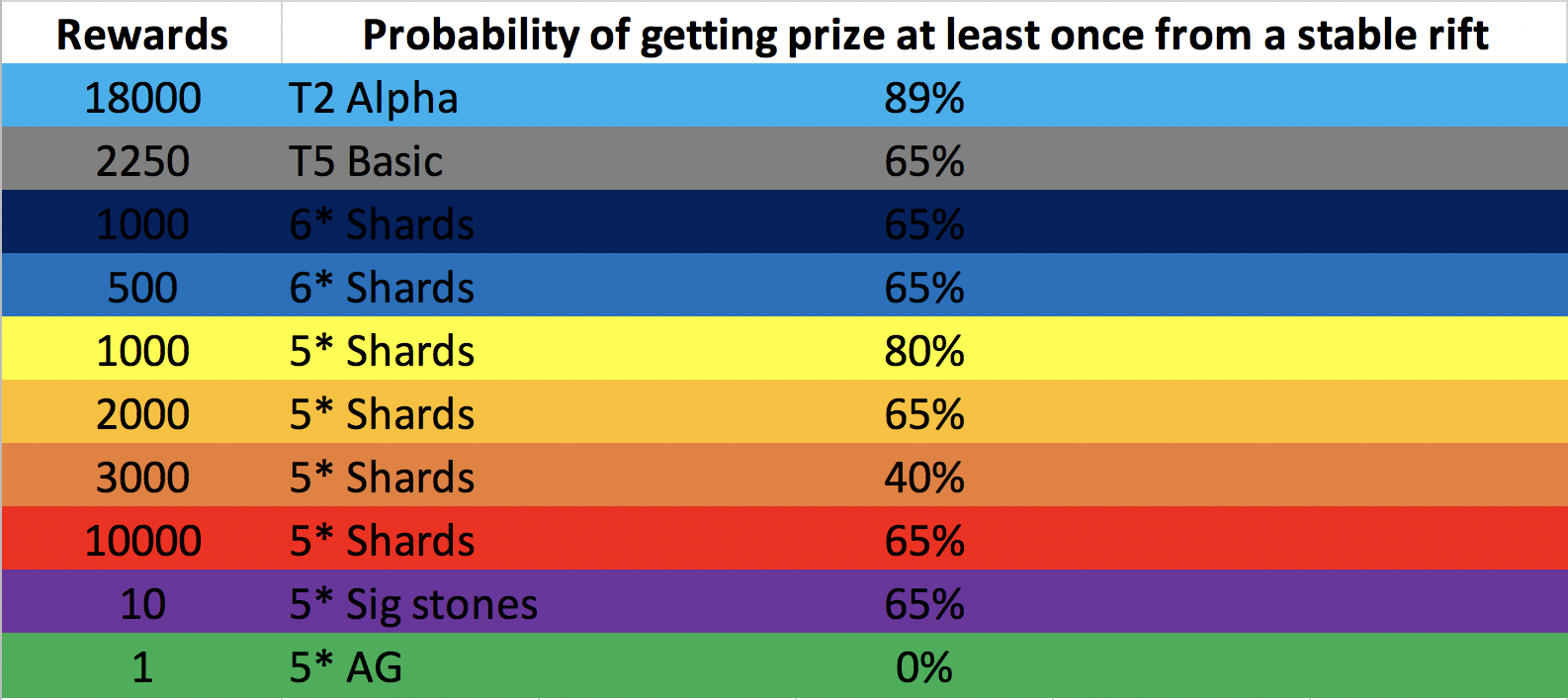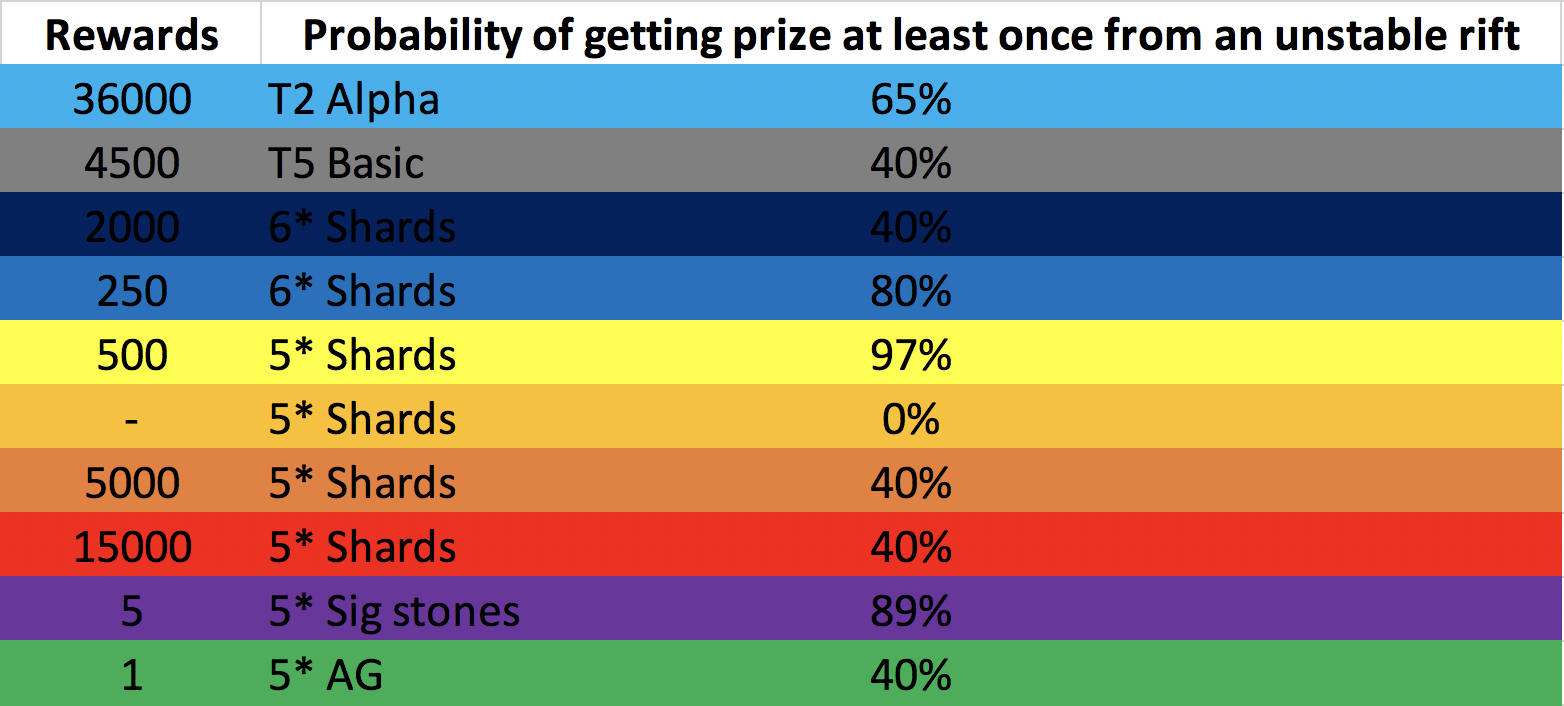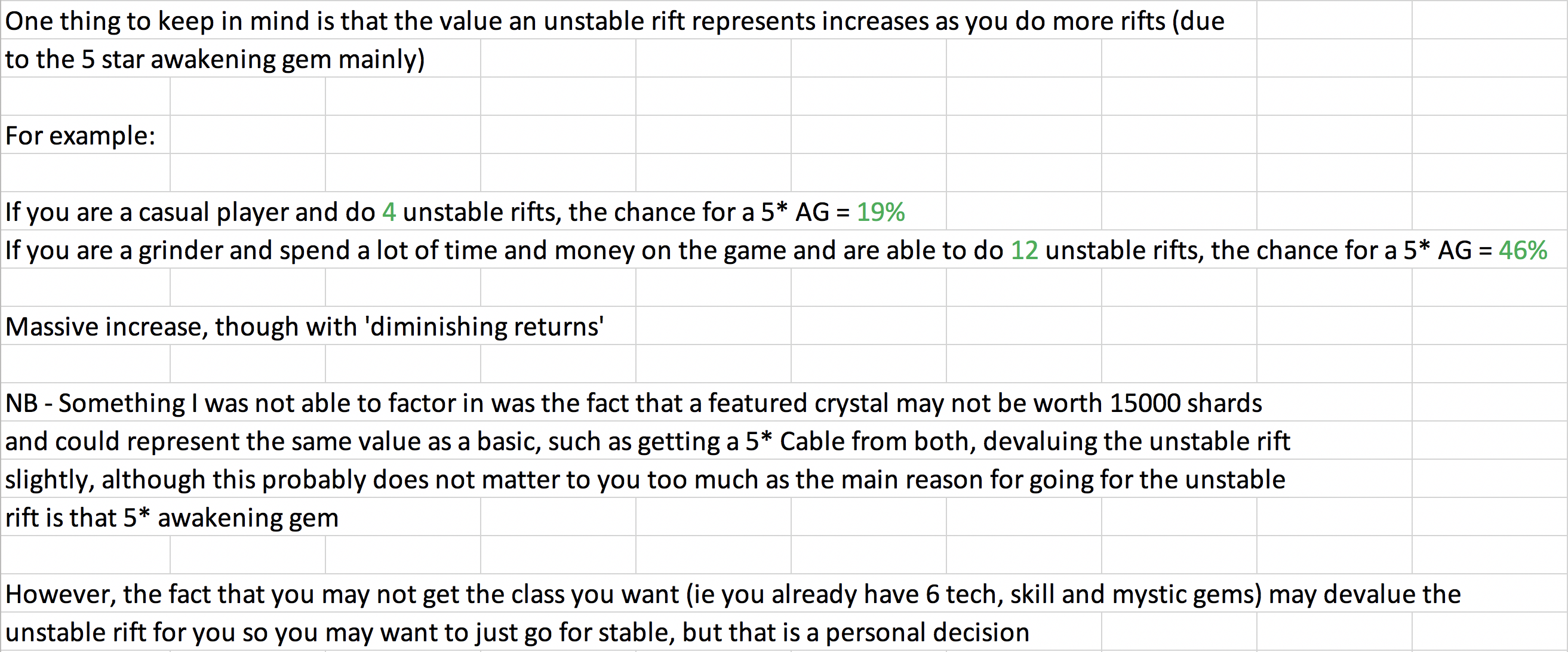«1

• whoa lots of words...
• Thanks man! Good to see there are such helpful people out there
• Soooo... we should go for the Unstable?
• What the... Thank you very much for your effort!
• Soooo... we should go for the Unstable?

Yes I think so, as long as you already have a decent number of 5 star champions, say 20 (otherwise you will really feel the shard loss)
• Thank you, this is awesome!
• Very helpful, I was looking something like it
• Only flaw in this thinking is the fact that right now the max is 8 assuming you rank #1 in every f4 hero use. (A more realistic number is 5) although i cant 100% Uc so probably more like 4 for me. There will be 2 more from ways unknown. Hopefully not purchasing but there is a very large chance of that.
• Only flaw in this thinking is the fact that right now the max is 8 assuming you rank #1 in every f4 hero use. (A more realistic number is 5) although i cant 100% Uc so probably more like 4 for me. There will be 2 more from ways unknown. Hopefully not purchasing but there is a very large chance of that.

Yeah I think richtheman said 8 max based on current situation, but I guess there will be some unit offers coming up and probably a few other ways to bump up to 10 I imagine. If you only do 8 though, the probability of getting an awakening gem at least once is 34%, so approximately 1/3 so I'd say unstable would still be worth it. By the way, I've scored around 700k the last couple of times, and this has put me in the top 1%-20% in solo events in case anyone was wondering.
• Hey man so what would be the probability of getting an awakening gem if someone could only do 8 rifts?
• Hey man so what would be the probability of getting an awakening gem if someone could only do 8 rifts?

Hey, good question. It's looking likely that without overspending or excessive grinding that the maximum number of rifts you could actually complete would be about 8. The probability of pulling at least one 5 star awakening gem from 8 unstable rifts would be around 34%. Interestingly, this is only a 6% decrease from doing 10 rifts. This means that you reduce your chance of pulling a 5star AG to about 1/3 - I'd personally recommend anyone with a decent number of 5 stars to roll with those odds, considering I pulled 2 science awakening gems back to back from crystals - a probability of only 2.6% - 1 in 36!
• Hey man so what would be the probability of getting an awakening gem if someone could only do 8 rifts?

It is a 5 percent drop rate so the appropriate calculation for 8 runs is 1-(0.95^8) which is approximately a 33.6% chance to get at least one awakening gem from 8 runs
• I got the T5 basics, but I really wanted the Skill AG. Can't complain since I lucked out on the 5% chance reward
• Verzz said:

Hey man so what would be the probability of getting an awakening gem if someone could only do 8 rifts?

It is a 5 percent drop rate so the appropriate calculation for 8 runs is 1-(0.95^8) which is approximately a 33.6% chance to get at least one awakening gem from 8 runs
I dont think it works like that. Each individual run will trigger a 5% chance for the AG, so its definitely a lot less than 33.6%.
• Verzz said:

Hey man so what would be the probability of getting an awakening gem if someone could only do 8 rifts?

It is a 5 percent drop rate so the appropriate calculation for 8 runs is 1-(0.95^8) which is approximately a 33.6% chance to get at least one awakening gem from 8 runs
I dont think it works like that. Each individual run will trigger a 5% chance for the AG, so its definitely a lot less than 33.6%.
It works exactly like that. Here's the way the math actually works out in long form.

Let's start by opening one crystal. The odds of getting the AG are 5%, which is another way of saying there's five out of one hundred chance to get the AG. So if you were to open a hundred crystals then on average you'd expect to get five AGs and 95 something else. Imagine a room full of players. All of them open one crystal. 5% of them get an AG, 95% don't (on average). Imagine all the winners leave that room and enter another room: the winners' room. So after opening one crystal 95% of the players are still in the original room. Now we open a second crystal. 5% of those players should get an AG (on average), and 95% should miss again. 5% of *those* players are now winners and leave the room. How many was that? Well, it was 5% of 95% of the original, which is 4.75% of the original. So the winners' room has 5% + 4.75% = 9.75% of the original players. Meanwhile, 95% of 95% are still in the original room, which is 90.25% of the original players.

See the pattern? First, there's 95% left (the "losers"). Then there's 95% x 95% = 90.25% left. Then there will be 95% x 95% x 95% = 85.74%. The "losers" are 95% x 95% x ... 95% N times after you open X crystals, on average. So if you open eight crystals, the number of players in the losers room will be 95% x 95% x 95% x 95% x 95% x 95% x 95% x 95% = 0.95 ^ 8 = 66.34% of the original players.

If 66.34% of the original players are still in the original (losers) room because they haven't pulled an AG, then that means 33.66% of the original players are in the winners' room because they did pull an AG at some point. The statistical formulas are mathematical short hand for doing this kind of counting.
• DNA3000 said:

Verzz said:

Hey man so what would be the probability of getting an awakening gem if someone could only do 8 rifts?

It is a 5 percent drop rate so the appropriate calculation for 8 runs is 1-(0.95^8) which is approximately a 33.6% chance to get at least one awakening gem from 8 runs
I dont think it works like that. Each individual run will trigger a 5% chance for the AG, so its definitely a lot less than 33.6%.
It works exactly like that. Here's the way the math actually works out in long form.

Let's start by opening one crystal. The odds of getting the AG are 5%, which is another way of saying there's five out of one hundred chance to get the AG. So if you were to open a hundred crystals then on average you'd expect to get five AGs and 95 something else. Imagine a room full of players. All of them open one crystal. 5% of them get an AG, 95% don't (on average). Imagine all the winners leave that room and enter another room: the winners' room. So after opening one crystal 95% of the players are still in the original room. Now we open a second crystal. 5% of those players should get an AG (on average), and 95% should miss again. 5% of *those* players are now winners and leave the room. How many was that? Well, it was 5% of 95% of the original, which is 4.75% of the original. So the winners' room has 5% + 4.75% = 9.75% of the original players. Meanwhile, 95% of 95% are still in the original room, which is 90.25% of the original players.

See the pattern? First, there's 95% left (the "losers"). Then there's 95% x 95% = 90.25% left. Then there will be 95% x 95% x 95% = 85.74%. The "losers" are 95% x 95% x ... 95% N times after you open X crystals, on average. So if you open eight crystals, the number of players in the losers room will be 95% x 95% x 95% x 95% x 95% x 95% x 95% x 95% = 0.95 ^ 8 = 66.34% of the original players.

If 66.34% of the original players are still in the original (losers) room because they haven't pulled an AG, then that means 33.66% of the original players are in the winners' room because they did pull an AG at some point. The statistical formulas are mathematical short hand for doing this kind of counting.
@Milan1405 This is what I was told is that attempts aren't cumulative.
if you run T4 Unstable 3x, you have a 5% chance to get the 5* AG, 3 times.

if you run T4 Unstable 1000x, you have a 5% chance to get the 5* AG, 1000 times
• he's looking at it like this (and in this example, i'm saying 1% chance vs the 5% chance that's given by Kabam):

You have a 99% chance to lose.

The chance you lose twice in a row is 99% or 99%, or .99*.99

The chance you lose three times in a row is .99 *.99 *.99.

The chance you lose 100 times in a row is .99 times itself 100 times

That comes to .36 or so.

So, if your chance to lose 100 in a row is .36, your chance to win at some point in that run is .64 (found by taking 1-.36)

that's how he's getting a 40% chance to get a 5* AG from running T4 Unstable 10x.
• Verzz said:

Hey man so what would be the probability of getting an awakening gem if someone could only do 8 rifts?

It is a 5 percent drop rate so the appropriate calculation for 8 runs is 1-(0.95^8) which is approximately a 33.6% chance to get at least one awakening gem from 8 runs
I dont think it works like that. Each individual run will trigger a 5% chance for the AG, so its definitely a lot less than 33.6%.
You are wrong - please read DNA’s point as I can’t break it down more than he did for this exact situation. It is just formula that makes calculating odds easier which is 1-(X^Y) where X is the odds of something hitting (or not hitting depending how you are looking at it) expressed as a decimal and Y is the number of chances you have.

In this case X is 0.95 because is the chance of it not being the gem and Y is 8 tries. Sorry I know it is confusing but it is hard to try to explain this on my phone
• Also slightly related but more probably a brag post - I got the gem on my first run so if I still have 7 more I still have 1-(.95^7)= over 30% chance to get another one! Woohoo
• ^^Close but not quite! In this situation you use something called a Markov chain. This is a statistical approach, where the outcome (ie you getting an awakening gem on an attempt) is based on the present state to determine a future one, but not on any previous attempts, using matricies. This is quite a complicated area of mathematics and computer science, but if you are interested there is a lot to learn about them on google - which, coincidentally, is modelled as a Markov chain!
• Milan1405 said:

^^Close but not quite! In this situation you use something called a Markov chain. This is a statistical approach, where the outcome (ie you getting an awakening gem on an attempt) is based on the present state to determine a future one, but not on any previous attempts, using matricies. This is quite a complicated area of mathematics and computer science, but if you are interested there is a lot to learn about them on google - which, coincidentally, is modelled as a Markov chain!

You would never use a Markov chain for this calculation, because it reduces to a trivial chain if you analyze for next opening probability, and arguably non-Markovian if you analyze for output value.

Where you might use a Markov chain is to analyze a situation like this: I save 5* shards until the next featured crystal arrives at a rate of 20k per week. I will open featured crystals until I get a featured champ, then open basic crystals until I run out of shards, unless I awaken a god tier champ, in which case I will stop until the next featured crystal arrives. What's the average number of shards I'm likely to have at any moment in time over the long run? How often will I run out?

Google isn't modelled as a Markov chain, that's kind of a weird statement. I believe you're referring to the Pagemark algorithm which forms the original heart of the Google system. Pagemark models people as random web browsers that read a page, randomly click on a link on that page to go to another page, and repeat until they get bored and restart the process on another random page. In other words, tvtropes and wikipedia hell. This can be analyzed as a stochastic Markov process to find steady state transition vectors that assign weights to every web page that can be used to measure how likely a person would be to find and read the page, and use that as a measure of "importance." Important pages are those that more people read, or more web authors link to. Pagemark maps the problem of finding a solution to the problem of assigning page weights to a Markovian analysis.

See: http://blog.kleinproject.org/?p=280
• DNA3000 said:

Milan1405 said:

^^Close but not quite! In this situation you use something called a Markov chain. This is a statistical approach, where the outcome (ie you getting an awakening gem on an attempt) is based on the present state to determine a future one, but not on any previous attempts, using matricies. This is quite a complicated area of mathematics and computer science, but if you are interested there is a lot to learn about them on google - which, coincidentally, is modelled as a Markov chain!

You would never use a Markov chain for this calculation, because it reduces to a trivial chain if you analyze for next opening probability, and arguably non-Markovian if you analyze for output value.

Where you might use a Markov chain is to analyze a situation like this: I save 5* shards until the next featured crystal arrives at a rate of 20k per week. I will open featured crystals until I get a featured champ, then open basic crystals until I run out of shards, unless I awaken a god tier champ, in which case I will stop until the next featured crystal arrives. What's the average number of shards I'm likely to have at any moment in time over the long run? How often will I run out?

Google isn't modelled as a Markov chain, that's kind of a weird statement. I believe you're referring to the Pagemark algorithm which forms the original heart of the Google system. Pagemark models people as random web browsers that read a page, randomly click on a link on that page to go to another page, and repeat until they get bored and restart the process on another random page. In other words, tvtropes and wikipedia hell. This can be analyzed as a stochastic Markov process to find steady state transition vectors that assign weights to every web page that can be used to measure how likely a person would be to find and read the page, and use that as a measure of "importance." Important pages are those that more people read, or more web authors link to. Pagemark maps the problem of finding a solution to the problem of assigning page weights to a Markovian analysis.

See: http://blog.kleinproject.org/?p=280
Thanks for the information as usual. Just out of curiosity how much of a pain is it to do that calculation that you talked about in your example of 20k shards per week etc?
• @DNA3000 there is a new quantum branch of markov chains that does sort of look at this thing (don't ask me how it works lol) but yeh technically google isn't a markov chain its more to do with modelling the world wide web and page rank computation but people get lost when we delve into stuff this complicated without giving a simplified overview. So in short you are correct! I'd be happy for you add me on line - my name is Milan Patel so we can continue this discussion. It's very interesting and you are obviously a learned individual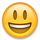• All I know is I got super lucky
•• Big_420 said:

All I know is I got super lucky

Congrats!
• Milan1405 said:

@DNA3000 there is a new quantum branch of markov chains that does sort of look at this thing (don't ask me how it works lol) but yeh technically google isn't a markov chain its more to do with modelling the world wide web and page rank computation but people get lost when we delve into stuff this complicated without giving a simplified overview. So in short you are correct! I'd be happy for you add me on line - my name is Milan Patel so we can continue this discussion. It's very interesting and you are obviously a learned individualYeah @Milan1405 @DNA3000 my friend is working in quantum markov chain realm and he started telling me about how you could model this problem using a very new and not very understood area of maths/physics at the moment - then I got lost lol. He started talking about eigenvectors etc. Also appreciate the information you guys give out as there is a lot of fake news out on areas of quantum and its also easy to misinterpret. Also Milan I appreciate the effort to simplify this content but its okay to explain it in detail and people who want to learn more can just look it up so I'd rather that than oversimplification but thanks from myself and im sure the rest of the community for the clear breakdown and tables dude!
• Verzz said:

DNA3000 said:

Milan1405 said:

^^Close but not quite! In this situation you use something called a Markov chain. This is a statistical approach, where the outcome (ie you getting an awakening gem on an attempt) is based on the present state to determine a future one, but not on any previous attempts, using matricies. This is quite a complicated area of mathematics and computer science, but if you are interested there is a lot to learn about them on google - which, coincidentally, is modelled as a Markov chain!

You would never use a Markov chain for this calculation, because it reduces to a trivial chain if you analyze for next opening probability, and arguably non-Markovian if you analyze for output value.

Where you might use a Markov chain is to analyze a situation like this: I save 5* shards until the next featured crystal arrives at a rate of 20k per week. I will open featured crystals until I get a featured champ, then open basic crystals until I run out of shards, unless I awaken a god tier champ, in which case I will stop until the next featured crystal arrives. What's the average number of shards I'm likely to have at any moment in time over the long run? How often will I run out?

Google isn't modelled as a Markov chain, that's kind of a weird statement. I believe you're referring to the Pagemark algorithm which forms the original heart of the Google system. Pagemark models people as random web browsers that read a page, randomly click on a link on that page to go to another page, and repeat until they get bored and restart the process on another random page. In other words, tvtropes and wikipedia hell. This can be analyzed as a stochastic Markov process to find steady state transition vectors that assign weights to every web page that can be used to measure how likely a person would be to find and read the page, and use that as a measure of "importance." Important pages are those that more people read, or more web authors link to. Pagemark maps the problem of finding a solution to the problem of assigning page weights to a Markovian analysis.

See: http://blog.kleinproject.org/?p=280
Thanks for the information as usual. Just out of curiosity how much of a pain is it to do that calculation that you talked about in your example of 20k shards per week etc?
If linear algebra is your hobby, it doesn't take much work to do the actual calculation. The work comes in setting up the assumptions and conditions behind the calculation. I'd probably cheat and do a Monte Carlo (pseudo) Markov python script.

The basic idea is that it can be difficult to properly express all the conditions and transitions in equation or matrix form. But it can be easier to express them algorithmically. So you write a program (or script) that basically goes step by step and simulates opening crystals and burning shards over a period of simulated time, all the while adding crystals, adding pretend champions to the game, etc. That's just step by step lines of code. Then you run it a million times and average the results. That will give you an estimate for what the actual values are in steady state.

An example of the kind of analysis I'd use Monte Carlo Markov for is comparing the signature ability of Red Magneto and White Magneto. Red Magneto has a safeguard that is always on while White Magneto has a safeguard that only triggers when low health but is stronger than Red Magneto's. So which one is better? Well, they are different so there's no one correct answer, but you can ask questions to try to compare them, like "given certain reasonable assumptions about the strength of the attacker, which one is harder/takes longer to kill?" And that's something that tends to be easier to solve by Monte Carlo Markov analysis. Especially because if you make a mistake or want to redo the analysis with different assumptions, instead of doing a whole bunch of math again you just tweak some numbers and rerun your script, and grab a beer while you wait.
• DNA3000 said:

Verzz said:

DNA3000 said:

Milan1405 said:

^^Close but not quite! In this situation you use something called a Markov chain. This is a statistical approach, where the outcome (ie you getting an awakening gem on an attempt) is based on the present state to determine a future one, but not on any previous attempts, using matricies. This is quite a complicated area of mathematics and computer science, but if you are interested there is a lot to learn about them on google - which, coincidentally, is modelled as a Markov chain!

You would never use a Markov chain for this calculation, because it reduces to a trivial chain if you analyze for next opening probability, and arguably non-Markovian if you analyze for output value.

Where you might use a Markov chain is to analyze a situation like this: I save 5* shards until the next featured crystal arrives at a rate of 20k per week. I will open featured crystals until I get a featured champ, then open basic crystals until I run out of shards, unless I awaken a god tier champ, in which case I will stop until the next featured crystal arrives. What's the average number of shards I'm likely to have at any moment in time over the long run? How often will I run out?

Google isn't modelled as a Markov chain, that's kind of a weird statement. I believe you're referring to the Pagemark algorithm which forms the original heart of the Google system. Pagemark models people as random web browsers that read a page, randomly click on a link on that page to go to another page, and repeat until they get bored and restart the process on another random page. In other words, tvtropes and wikipedia hell. This can be analyzed as a stochastic Markov process to find steady state transition vectors that assign weights to every web page that can be used to measure how likely a person would be to find and read the page, and use that as a measure of "importance." Important pages are those that more people read, or more web authors link to. Pagemark maps the problem of finding a solution to the problem of assigning page weights to a Markovian analysis.

See: http://blog.kleinproject.org/?p=280
Thanks for the information as usual. Just out of curiosity how much of a pain is it to do that calculation that you talked about in your example of 20k shards per week etc?
If linear algebra is your hobby, it doesn't take much work to do the actual calculation. The work comes in setting up the assumptions and conditions behind the calculation. I'd probably cheat and do a Monte Carlo (pseudo) Markov python script.

The basic idea is that it can be difficult to properly express all the conditions and transitions in equation or matrix form. But it can be easier to express them algorithmically. So you write a program (or script) that basically goes step by step and simulates opening crystals and burning shards over a period of simulated time, all the while adding crystals, adding pretend champions to the game, etc. That's just step by step lines of code. Then you run it a million times and average the results. That will give you an estimate for what the actual values are in steady state.

An example of the kind of analysis I'd use Monte Carlo Markov for is comparing the signature ability of Red Magneto and White Magneto. Red Magneto has a safeguard that is always on while White Magneto has a safeguard that only triggers when low health but is stronger than Red Magneto's. So which one is better? Well, they are different so there's no one correct answer, but you can ask questions to try to compare them, like "given certain reasonable assumptions about the strength of the attacker, which one is harder/takes longer to kill?" And that's something that tends to be easier to solve by Monte Carlo Markov analysis. Especially because if you make a mistake or want to redo the analysis with different assumptions, instead of doing a whole bunch of math again you just tweak some numbers and rerun your script, and grab a beer while you wait.
Thank you for this - it was interesting and made sense so I will for sure look into it more myself. It is just not something I have heard of before as I do not have extensive studies or experience in this area